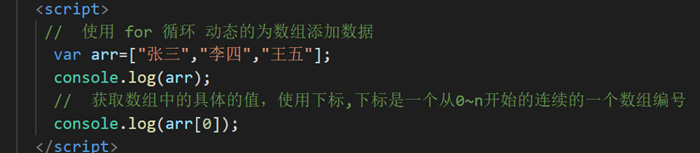# JavaScript如何创建数组？

## 如何定义创建数组？

//使用[]来定义数组
//1.先创建一个空数组  然后再往这个空数组里面添加数组元素 通过下标来添加
// 数组变量名[下标] = 值
var arr1 = []; //没有数组元素就称之为空数组
arr1 = "鸣人";
arr1 = "一护";
arr1 = "翠花";
arr1 = "卡卡罗特";
//获取数组的长度  一个变量没有赋值
console.log(arr1);

<script>
//使用[]来定义数组
//1.先创建一个空数组  然后再往这个空数组里面添加数组元素 通过下标来添加
// 数组变量名[下标] = 值
var arr1 = []; //没有数组元素就称之为空数组
arr1 = "鸣人";
arr1 = "一护";
arr1 = "翠花";
arr1 = "卡卡罗特";
//获取数组的长度  一个变量没有赋值
console.log(arr1);
//第二种方式：在定义数组时候直接添加数组元素
var arr2 = ['佐助','鸣人','小樱',18];
console.log(arr2);

</script>

<script>
//先定义空数组
//使用 for 循环 动态的为数组添加数据
var arr=[];
for(var i=0;i<10;i++){
arr[i]=i+"哈哈哈";
}
console.log(arr);
</script><script>
//使用new关键字和Array()方法来定义数组
//1.创建一个空数组  new Array();

var arr1 = new Array();   // new Date()
arr1 = "孙悟空";
arr1 = "花果山";
arr1 = "方寸山斜月三星洞";

//2.创建一个有指定长度的数组
//也就是数组的元素有三个 想写4个 或者只写2个
//规定了长度 但是少写了 数组的长度还是规定的
var arr2 = new Array(3);  //数组的长度是3  也就是数组的元素有三个
//添加数组元素
arr2 = "猪刚鬣";
arr2 = "高老庄";
arr2 = "好色";
arr2 = "好吃";

console.log(arr2.length);

//3.创建一个数组 数组里面有指定的元素
var arr3 = new Array("鸣人",18,"木叶","雏田");
console.log(arr3);

</script>

## 数组的相关概念

`var arr=[“张三”,”李四”,”王五”];`

`数组变量名[下标]``数组变量名.length`

<script>
//使用一个变量来保存所有同学的姓名
//数组在其它的程序语言里面是是数组类型 但是在JS里面没有数组类型 它是对象类型
//数组是一组数组的有序的集体  每个数据之间使用逗号分开 为了表示它是数组 要在这些数据的外面使用[]括起来
//数组是一组数据有序的集合 有顺序
//数组的长度 ----->数组里面的元素的个数
//语法：
//数组里面的元素它的类型可以是任意类型 没有限制
var arr =  ["张三","李四","王五",18,true,false];

//数组的下标的目的是为了让我们的找到数组里面的元素
//格式： 数组的变量名[指定的下标值]

console.log(arr);
console.log(arr);
//获取数组的长度 数组里面元素的总个数  JS仿照JAVA
console.log(arr.length);
//如果的最大下标与长度之间的关系
//数组里面最大的下标 = 数组的长度-1
var length = arr.length; //获取当前数组的长度将它赋值给变量length
console.log(arr[length-1]);

</script>

Javascript预解析详解

JavaScript中如何搜索数组元素?

## 相关阅读